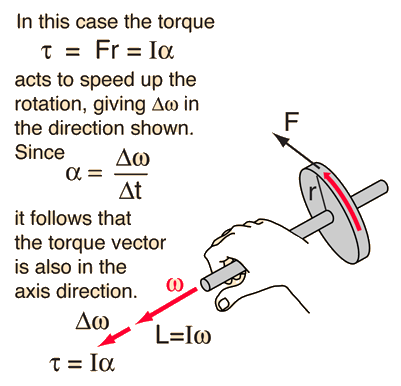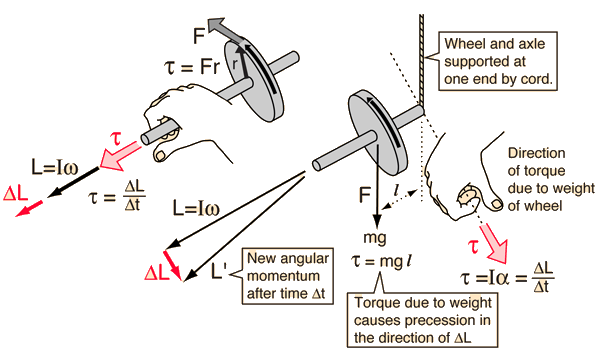# Rotation VectorsAngular motion has direction associated with it and is inherently a vector process. But a point on a rotating wheel is continuously changing direction and it is inconvenient to track that direction. The only fixed, unique direction for a rotating wheel is the axis of rotation, so it is logical to choose this axis direction as the direction of the angular velocity. Left with two choices about direction, it is customary to use the right hand rule to specify the direction of angular quantities.

 Direction of other angular quantities Vector rotation examples
Index

 HyperPhysics***** Mechanics ***** Rotation R Nave
Go Back

# Directions of Angular QuantitiesAs an example of the directions of angular quantities, consider a vector angular velocity as shown. If a force acts tangential to the wheel to speed it up, it follows that the change in angular velocity and therefore the angular acceleration are in the direction of the axis. Newton's 2nd law for rotation implies that the torque is also in the axis direction. The angular momentum will also be in this direction, so in this example, all of these angular quantities act along the axis of rotation as shown.

### What if the torque is perpendicular to the angular momentum?

Index

 HyperPhysics***** Mechanics ***** Rotation R Nave
Go Back

# Angular Momentum Change

A force tangential to the wheel produces a torque along the axis as shown (right hand rule). The change in angular momentum is therefore along the axis and the wheel increases in angular velocity. However, if the torque direction is perpendicular to the axis of the wheel the effect is very different. The change in angular velocity is perpendicular to the angular velocity vector, changing its direction but not its magnitude. The resultant motion of the wheel around a vertical axis is called precession.Index

 HyperPhysics***** Mechanics ***** Rotation R Nave
Go Back